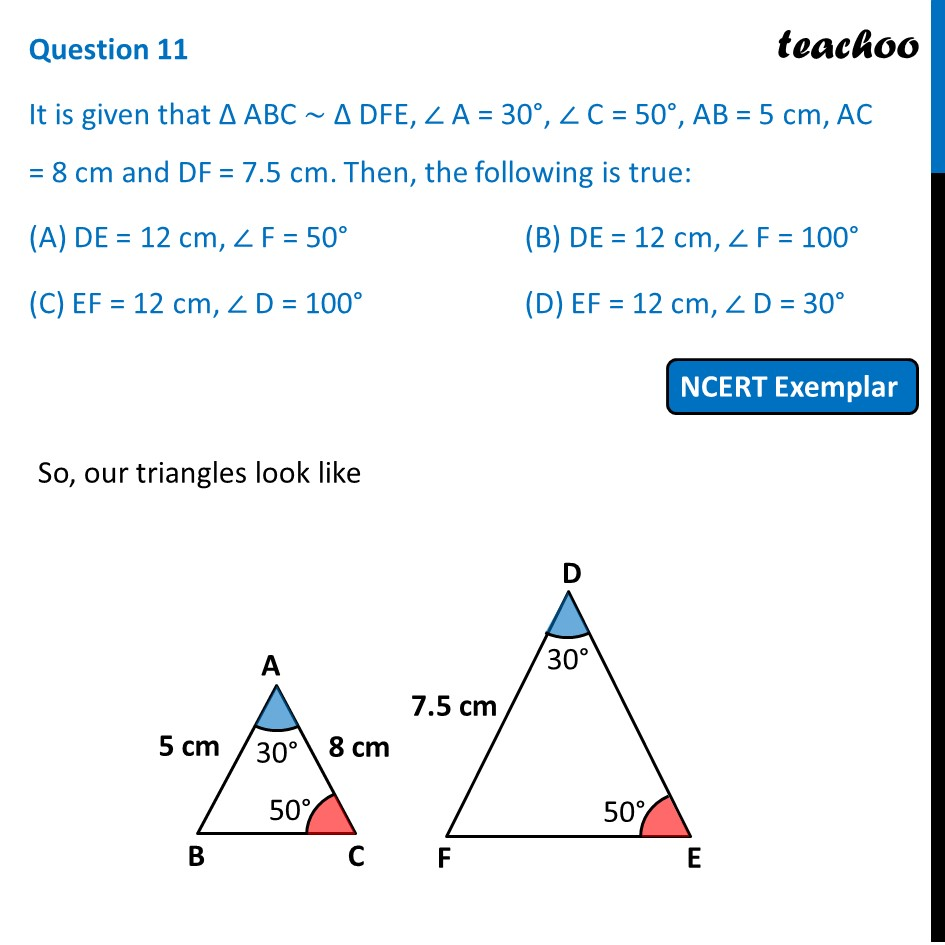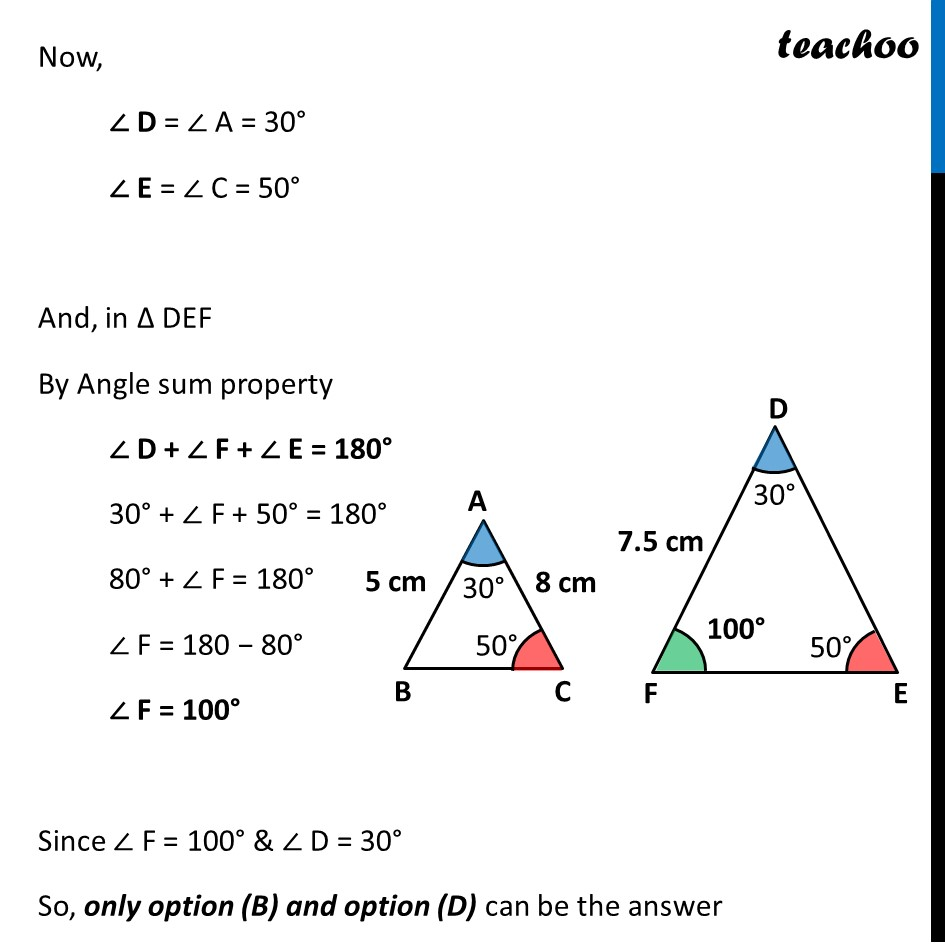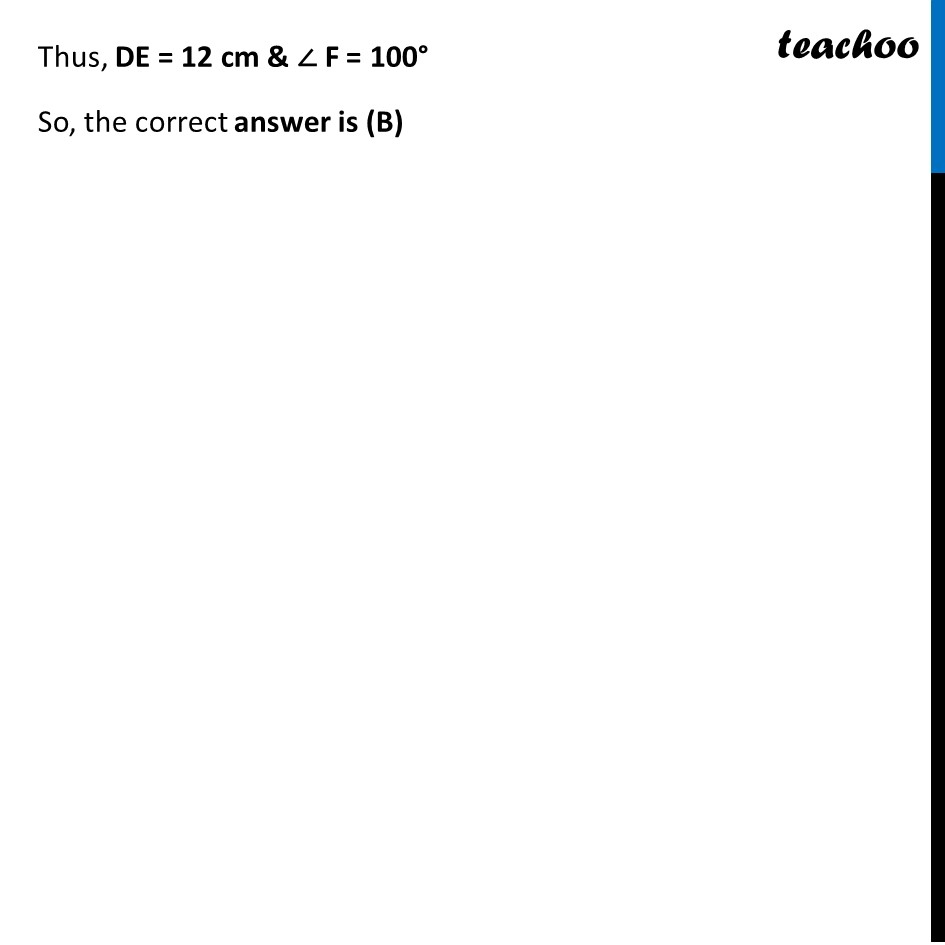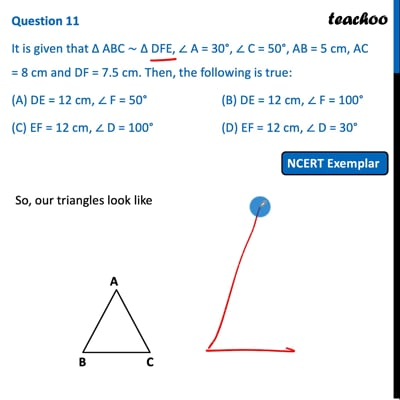NCERT Exemplar - MCQ

Chapter 6 Class 10 Triangles
Serial order wise

## (D) EF = 12 cm, ∠ D = 30°This video is only available for Teachoo black users

Maths Crash Course - Live lectures + all videos + Real time Doubt solving!

### Transcript

Question 11 It is given that ∆ ABC ~ ∆ DFE, ∠ A = 30°, ∠ C = 50°, AB = 5 cm, AC = 8 cm and DF = 7.5 cm. Then, the following is true: (A) DE = 12 cm, ∠ F = 50° (B) DE = 12 cm, ∠ F = 100° (C) EF = 12 cm, ∠ D = 100° (D) EF = 12 cm, ∠ D = 30° So, our triangles look like Now, ∠ D = ∠ A = 30° ∠ E = ∠ C = 50° And, in Δ DEF By Angle sum property ∠ D + ∠ F + ∠ E = 180° 30° + ∠ F + 50° = 180° 80° + ∠ F = 180° ∠ F = 180 − 80° ∠ F = 100° Since ∠ F = 100° & ∠ D = 30° So, only option (B) and option (D) can be the answer Since ∆ ABC ~ ∆ DFE Corresponding sides are proportional ∴ 𝐴𝐵/𝐷𝐹=𝐴𝐶/𝐷𝐸=𝐵𝐶/𝐸𝐹 Putting values 𝟓/(𝟕.𝟓)=𝟖/𝑫𝑬=𝑩𝑪/𝑬𝑭 Comparing 𝟓/(𝟕.𝟓)=𝟖/𝑫𝑬 2/3=8/𝐷𝐸 DE = 8 × 3/2 DE = 12 cm Thus, DE = 12 cm & ∠ F = 100° So, the correct answer is (B)